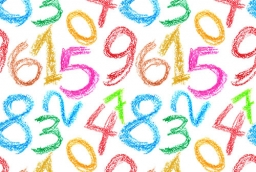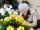# Seven numbers

Write seven 4-digit numbers that are divisible by 3 and at the same time by 4.

a1 =  1008
a2 =  1020
a3 =  1032
a4 =  1044
a5 =  1056
a6 =  1068
a7 =  1080

### Step-by-step explanation:Did you find an error or inaccuracy? Feel free to write us. Thank you!Tips to related online calculators
Do you want to calculate greatest common divisor two or more numbers?
Do you want to round the number?

## Related math problems and questions:

• Common divisorsFind all common divisors of numbers 30 and 45.
• Three friendsThree friends had balls in ratio 2: 7: 4 at the start of the game. Could they have the same number of balls at the end of the game? Write 0, if not, or write the minimum number of balls they had together.
• LCD 2The least common denominator of 2/5, 1/2, and 3/4
• DecomposeDecompose into primes and find the smallest common multiple n of (16,20) and the largest common divisor D of the pair of numbers (140,100)
• MO C–I–1 2018An unknown number is divisible by just four numbers from the set {6, 15, 20, 21, 70}. Determine which ones.
• FloristFlorist has 84 red and 48 white roses. How many same bouquets can he make from them if he must use all roses?
• Divisible by nineHow many three-digit natural numbers in total are divisible without a remainder by the number 9?
• GroupsIn the 6th class there are 60 girls and 72 boys. We want to divide them into groups so that the number of girls and boys is the same. How many groups can you create? How many girls will be in the group?
• Simplest form 3What fraction is 15 of 35 in simplest form?
• 6/24 change6/24 change to a fraction in lowest terms
• QuotientFind quotient before the bracket - the largest divisor 51 a + 34 b + 68 121y-99z-331st blade 2,5 m, 2nd blade. .1.75 m. How many same long pieces of this two blades can be do the biggest? How long is one piece?There are given the number C = 281, D = 201. Find the highest natural number S so that the C:S, D:S are with the remainder of 1,The local reader’s club has a set of 28 hardback books and a set of 44 paperbacks. Each set can be divided equally among the club members. What is the greatest possible number of club members?Anna thinks of a five-digit number that is not divisible by three or four. If he increments each digit by one, it gets a five-digit number that is divisible by three. If he reduces each digit by one, he gets a five-digit number divisible by four. If it swThere are four trees in the alley between which the distances are 35m, 15m and 95m. Trees must be laid in the spaces so that the distance is the same and the maximum. How many trees will they put in and what will be the distance between them?From two sticks 240 cm and 210 cm long, it is necessary to cut the longest possible pegs for flowers so that no residues remain. How many pegs will it be?